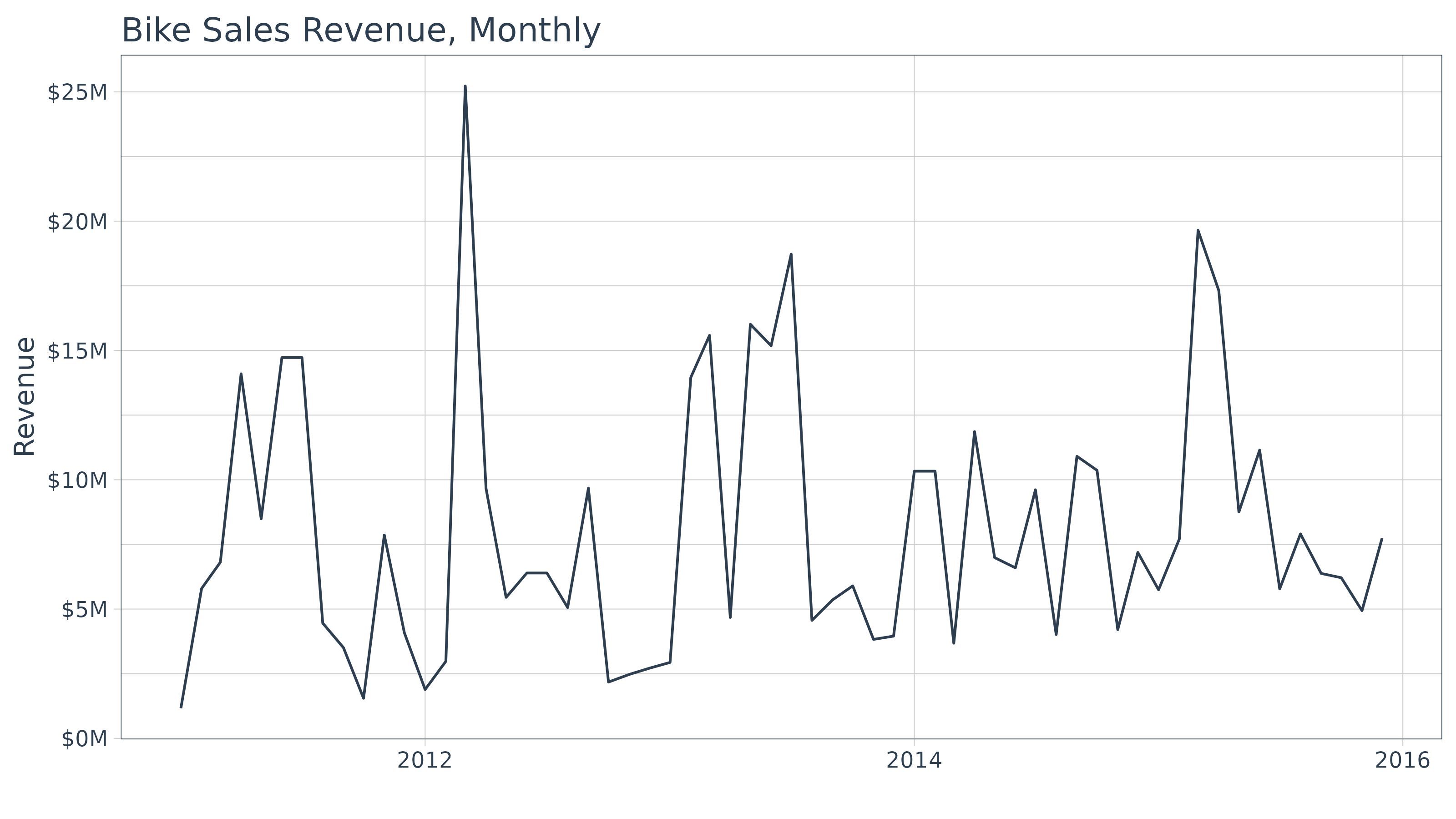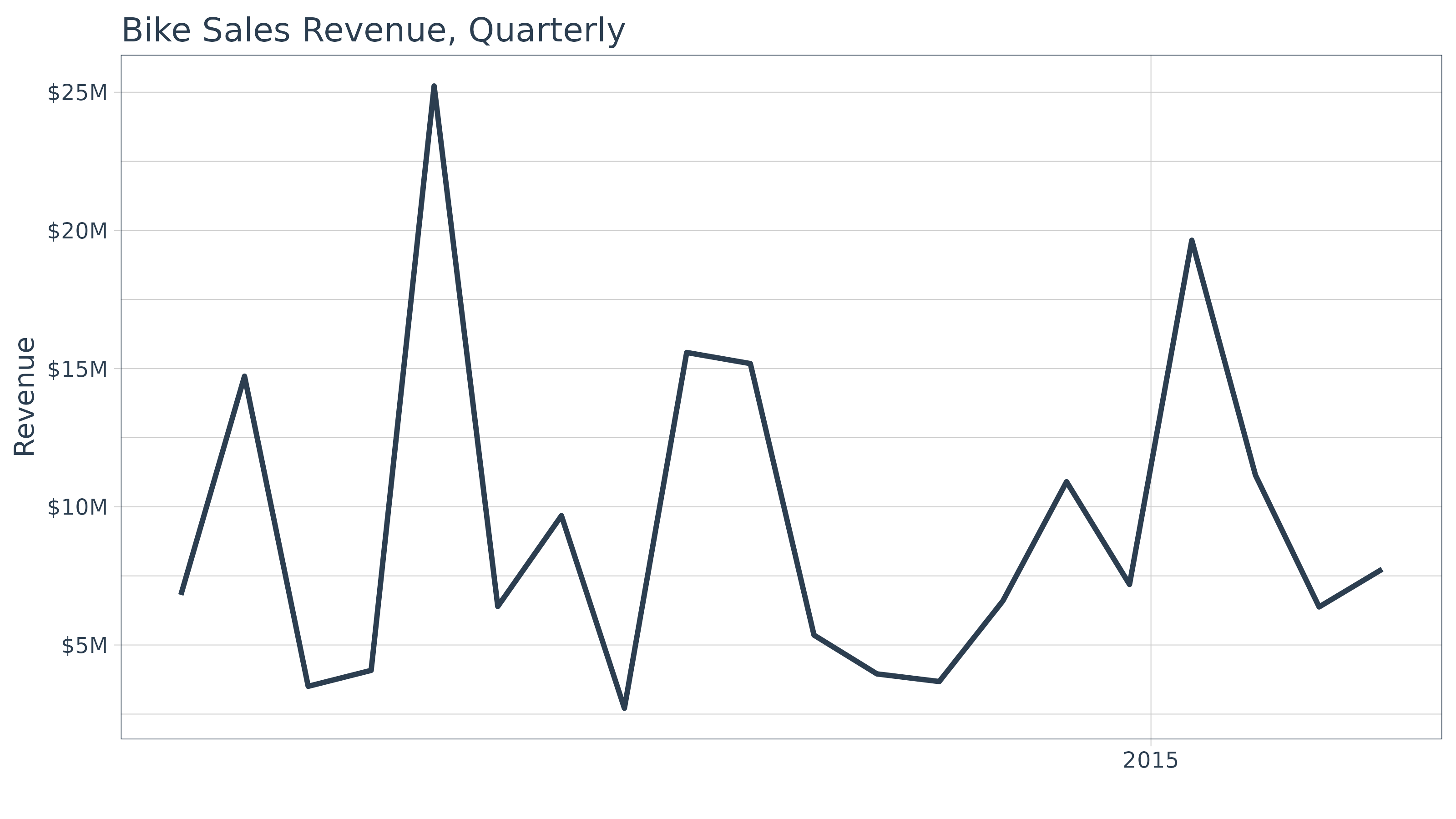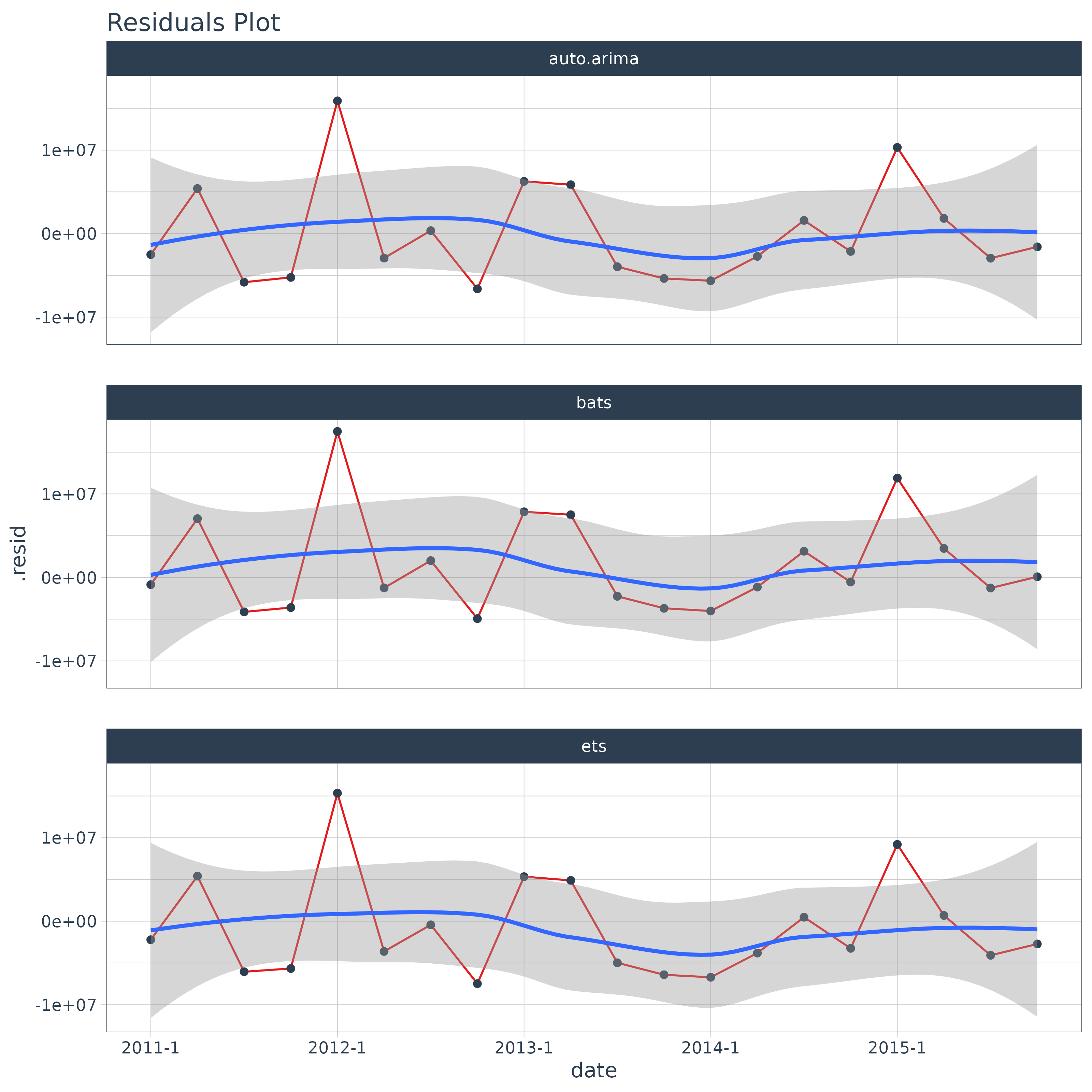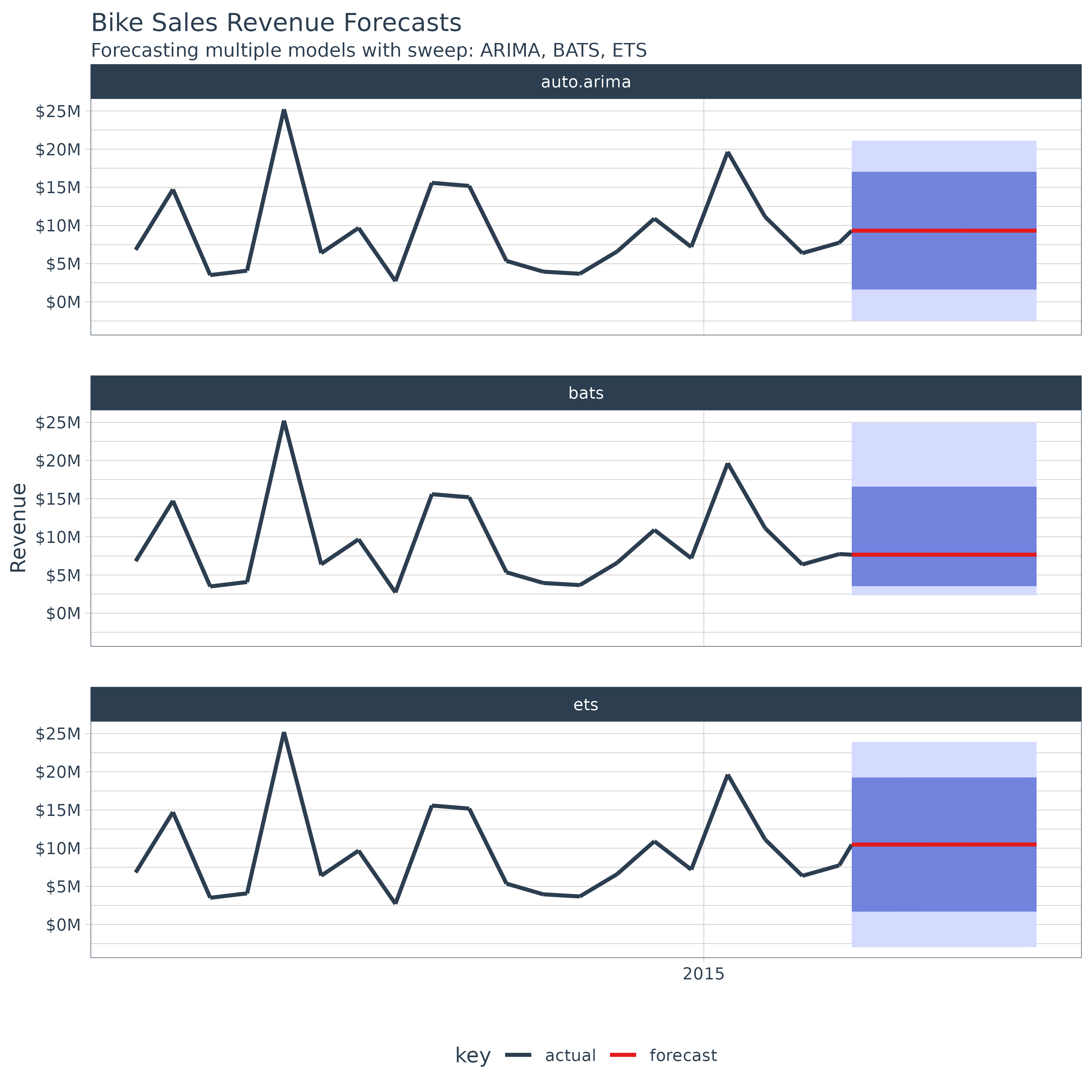Extending broom to time series forecasting

One of the most powerful benefits of sweep is that it helps forecasting at scale within the “tidyverse”. There are two common situations:

1. Applying a model to groups of time series
2. Applying multiple models to a time series

In this vignette we’ll review how sweep can help the second situation: Applying multiple models to a time series.

## Prerequisites

Before we get started, load the following packages.

library(tidyverse)
## Warning: package 'readr' was built under R version 4.0.5
library(tidyquant)
## Warning: package 'xts' was built under R version 4.0.5
## Warning: package 'zoo' was built under R version 4.0.5
## Warning: package 'TTR' was built under R version 4.0.5
library(timetk)
library(sweep)
library(forecast)
## Warning: package 'forecast' was built under R version 4.0.5

## Forecasting Gasoline Prices

To start, let’s get some data from the FRED data base using tidyquant. We’ll use tq_get() to retrieve the Gasoline Prices from 1990 through today (2023-07-04).

gas_prices_monthly_raw <- tq_get(
x    = "GASREGCOVM",
get  = "economic.data",
from = "1990-01-01",
to   = "2016-12-31")
gas_prices_monthly_raw
## # A tibble: 316 x 3
##    symbol     date       price
##    <chr>      <date>     <dbl>
##  1 GASREGCOVM 1990-09-01  1.26
##  2 GASREGCOVM 1990-10-01  1.34
##  3 GASREGCOVM 1990-11-01  1.32
##  4 GASREGCOVM 1990-12-01 NA
##  5 GASREGCOVM 1991-01-01 NA
##  6 GASREGCOVM 1991-02-01  1.09
##  7 GASREGCOVM 1991-03-01  1.04
##  8 GASREGCOVM 1991-04-01  1.08
##  9 GASREGCOVM 1991-05-01  1.13
## 10 GASREGCOVM 1991-06-01  1.13
## # i 306 more rows

Upon a brief inspection, the data contains 2 NA values that will need to be dealt with.

summary(gas_prices_monthly_raw$price) ## Min. 1st Qu. Median Mean 3rd Qu. Max. NA's ## 0.900 1.138 1.615 1.974 2.697 4.002 2 We can use the fill() from the tidyr package to help deal with these data. We first fill down and then fill up to use the previous and then post days prices to fill in the missing data. gas_prices_monthly <- gas_prices_monthly_raw %>% fill(price, .direction = "down") %>% fill(price, .direction = "up") We can now visualize the data. gas_prices_monthly %>% ggplot(aes(x = date, y = price)) + geom_line(color = palette_light()[]) + labs(title = "Gasoline Prices, Monthly", x = "", y = "USD") + scale_y_continuous(labels = scales::dollar) + theme_tq()Monthly periodicity might be a bit granular for model fitting. We can easily switch periodicity to quarterly using tq_transmute() from the tidyquant package along with the periodicity aggregation function to.period from the xts package. We’ll convert the date to yearqtr class which is regularized. gas_prices_quarterly <- gas_prices_monthly %>% tq_transmute(mutate_fun = to.period, period = "quarters") gas_prices_quarterly ## # A tibble: 106 x 2 ## date price ## <date> <dbl> ## 1 1990-09-01 1.26 ## 2 1990-12-01 1.32 ## 3 1991-03-01 1.04 ## 4 1991-06-01 1.13 ## 5 1991-09-01 1.11 ## 6 1991-12-01 1.08 ## 7 1992-03-01 1.01 ## 8 1992-06-01 1.14 ## 9 1992-09-01 1.12 ## 10 1992-12-01 1.08 ## # i 96 more rows Another quick visualization to show the reduction in granularity. gas_prices_quarterly %>% ggplot(aes(x = date, y = price)) + geom_line(color = palette_light()[], size = 1) + labs(title = "Gasoline Prices, Quarterly", x = "", y = "USD") + scale_y_continuous(labels = scales::dollar) + scale_x_date(date_breaks = "5 years", date_labels = "%Y") + theme_tq() ## Warning: Using size aesthetic for lines was deprecated in ggplot2 3.4.0. ## i Please use linewidth instead. ## This warning is displayed once every 8 hours. ## Call lifecycle::last_lifecycle_warnings() to see where this warning was ## generated.## Performing Forecasts Using Multiple Models In this section we will use three models to forecast gasoline prices: 1. ARIMA 2. ETS 3. BATS ### Multiple Models Concept Before we jump into modeling, let’s take a look at the multiple model process from R for Data Science, Chapter 25 Many Models. We first create a data frame from a named list. The example below has two columns: “f” the functions as text, and “params” a nested list of parameters we will pass to the respective function in column “f”. df <- tibble( f = c("runif", "rpois", "rnorm"), params = list( list(n = 10), list(n = 5, lambda = 10), list(n = 10, mean = -3, sd = 10) ) ) df ## # A tibble: 3 x 2 ## f params ## <chr> <list> ## 1 runif <named list > ## 2 rpois <named list > ## 3 rnorm <named list > We can also view the contents of the df$params column to understand the underlying structure. Notice that there are three primary levels and then secondary levels containing the name-value pairs of parameters. This format is important.

df$params ## [] ## []$n
##  10
##
##
## []
## []$n ##  5 ## ## []$lambda
##  10
##
##
## []
## []$n ##  10 ## ## []$mean
##  -3
##
## []$sd ##  10 Next we apply the functions to the parameters using a special function, invoke_map(). The parameter lists in the “params” column are passed to the function in the “f” column. The output is in a nested list-column named “out”. df_out <- df %>% mutate(out = invoke_map(f, params)) ## Warning: There was 1 warning in mutate(). ## i In argument: out = invoke_map(f, params). ## Caused by warning: ## ! invoke_map() was deprecated in purrr 1.0.0. ## i Please use map() + exec() instead. df_out ## # A tibble: 3 x 3 ## f params out ## <chr> <list> <list> ## 1 runif <named list > <dbl > ## 2 rpois <named list > <int > ## 3 rnorm <named list > <dbl > And, here’s the contents of “out”, which is the result of mapping a list of functions to a list of parameters. Pretty powerful! df_out$out
## []
##   0.7168111 0.3362430 0.2672394 0.2628689 0.2739463 0.3113810 0.2149605
##   0.7853907 0.1273967 0.8142541
##
## []
##  10  7  8  8 10
##
## []
##     0.9099061  -3.5457637  -3.2317158   9.2689360 -10.9789443   9.3618392
##    -6.9495087 -17.2391453 -15.9990590 -11.2272643

Take a minute to understand the conceptual process of the invoke_map function and specifically the parameter setup. Once you are comfortable, we can move on to model implementation.

### Multiple Model Implementation

We’ll need to take the following steps to in an actual forecast model implementation:

1. Coerce the data to time series
2. Build a model list using nested lists
3. Create the the model data frame
4. Invoke a function map

This is easier than it sounds. Let’s start by coercing the univariate time series with tk_ts().

gas_prices_quarterly_ts <- gas_prices_quarterly %>%
tk_ts(select = -date, start = c(1990, 3), freq = 4)
gas_prices_quarterly_ts
##       Qtr1  Qtr2  Qtr3  Qtr4
## 1990             1.258 1.324
## 1991 1.040 1.128 1.109 1.076
## 1992 1.013 1.145 1.122 1.078
## 1993 1.052 1.097 1.050 1.014
## 1994 1.008 1.078 1.144 1.060
## 1995 1.059 1.186 1.108 1.066
## 1996 1.129 1.243 1.200 1.233
## 1997 1.197 1.189 1.216 1.119
## 1998 1.014 1.048 0.994 0.923
## 1999 0.961 1.095 1.239 1.261
## 2000 1.498 1.612 1.525 1.418
## 2001 1.384 1.548 1.506 1.072
## 2002 1.221 1.341 1.363 1.348
## 2003 1.636 1.452 1.616 1.448
## 2004 1.689 1.910 1.841 1.800
## 2005 2.063 2.123 2.862 2.174
## 2006 2.413 2.808 2.501 2.284
## 2007 2.503 3.024 2.817 2.984
## 2008 3.215 3.989 3.709 1.669
## 2009 1.937 2.597 2.480 2.568
## 2010 2.742 2.684 2.678 2.951
## 2011 3.509 3.628 3.573 3.220
## 2012 3.774 3.465 3.801 3.256
## 2013 3.648 3.576 3.474 3.209
## 2014 3.474 3.626 3.354 2.488
## 2015 2.352 2.700 2.275 1.946
## 2016 1.895 2.303 2.161 2.192

Next, create a nested list using the function names as the first-level keys (this is important as you’ll see in the next step). Pass the model parameters as name-value pairs in the second level.

models_list <- list(
auto.arima = list(
y = gas_prices_quarterly_ts
),
ets = list(
y = gas_prices_quarterly_ts,
damped = TRUE
),
bats = list(
y = gas_prices_quarterly_ts
)
)

Now, convert to a data frame using the function, enframe() that turns lists into tibbles. Set the arguments name = "f" and value = "params". In doing so we get a bonus: the model names are the now convieniently located in column “f”.

models_tbl <- enframe(models_list, name = "f", value = "params")
models_tbl
## # A tibble: 3 x 2
##   f          params
##   <chr>      <list>
## 1 auto.arima <named list >
## 2 ets        <named list >
## 3 bats       <named list >

We are ready to invoke the map. Combine mutate() with invoke_map() as follows. Bada bing, bada boom, we now have models fitted using the parameters we defined previously.

models_tbl_fit <- models_tbl %>%
mutate(fit = invoke_map(f, params))
models_tbl_fit
## # A tibble: 3 x 3
##   f          params           fit
##   <chr>      <list>           <list>
## 1 auto.arima <named list > <fr_ARIMA>
## 2 ets        <named list > <ets>
## 3 bats       <named list > <bats>

## Inspecting the Model Fit

It’s a good point to review and understand the model output. We can review the model parameters, accuracy measurements, and the residuals using sw_tidy(), sw_glance(), and sw_augment().

### sw_tidy

The tidying function returns the model parameters and estimates. We use the combination of mutate and map to iteratively apply the sw_tidy() function as a new column named “tidy”. Then we unnest and spread to review the terms by model function.

models_tbl_fit %>%
mutate(tidy = map(fit, sw_tidy)) %>%
unnest(tidy) %>%
spread(key = f, value = estimate)
## # A tibble: 20 x 6
##    params           fit        term              auto.arima       bats       ets
##    <list>           <list>     <chr>                  <dbl>      <dbl>     <dbl>
##  1 <named list > <fr_ARIMA> ar1                    0.834 NA         NA
##  2 <named list > <fr_ARIMA> ma1                   -0.964 NA         NA
##  3 <named list > <fr_ARIMA> sar1                   0.939 NA         NA
##  4 <named list > <fr_ARIMA> sma1                  -0.776 NA         NA
##  5 <named list > <bats>     alpha                 NA      0.588     NA
##  6 <named list > <bats>     ar.coefficients       NA     NA         NA
##  7 <named list > <bats>     beta                  NA     NA         NA
##  8 <named list > <bats>     damping.parameter     NA     NA         NA
##  9 <named list > <bats>     gamma.values          NA     -0.0262    NA
## 10 <named list > <bats>     lambda                NA      0.0000605 NA
## 11 <named list > <bats>     ma.coefficients       NA      0.256     NA
## 12 <named list > <ets>      alpha                 NA     NA          0.831
## 13 <named list > <ets>      b                     NA     NA         -0.0524
## 14 <named list > <ets>      beta                  NA     NA          0.000100
## 15 <named list > <ets>      gamma                 NA     NA          0.0521
## 16 <named list > <ets>      l                     NA     NA          1.29
## 17 <named list > <ets>      phi                   NA     NA          0.837
## 18 <named list > <ets>      s0                    NA     NA          0.0469
## 19 <named list > <ets>      s1                    NA     NA         -0.0209
## 20 <named list > <ets>      s2                    NA     NA         -0.0407

### sw_glance

Glance is one of the most powerful tools because it yields the model accuracies enabling direct comparisons between the fit of each model. We use the same process for used for tidy, except theres no need to spread to perform the comparison. We can see that the ARIMA model has the lowest AIC by far.

models_tbl_fit %>%
mutate(glance = map(fit, sw_glance)) %>%
unnest(glance, .drop = TRUE)
## Warning: The .drop argument of unnest() is deprecated as of tidyr 1.0.0.
## i All list-columns are now preserved.
## Call lifecycle::last_lifecycle_warnings() to see where this warning was
## generated.
## # A tibble: 3 x 15
##   f     params       fit        model.desc sigma logLik   AIC   BIC     ME  RMSE
##   <chr> <list>       <list>     <chr>      <dbl>  <dbl> <dbl> <dbl>  <dbl> <dbl>
## 1 auto~ <named list> <fr_ARIMA> ARIMA(1,1~ 0.298  -20.6  51.2  64.4 0.0180 0.291
## 2 ets   <named list> <ets>      ETS(M,Ad,~ 0.118  -76.6 173.  200.  0.0149 0.292
## 3 bats  <named list> <bats>     BATS(0, {~ 0.116  159.  179.  184.  0.0193 0.259
## # i 5 more variables: MAE <dbl>, MPE <dbl>, MAPE <dbl>, MASE <dbl>, ACF1 <dbl>

### sw_augment

We can augment the models to get the residuals following the same procedure. We can pipe (%>%) the results right into ggplot() for plotting. Notice the ARIMA model has the largest residuals especially as the model index increases whereas the bats model has relatively low residuals.

models_tbl_fit %>%
mutate(augment = map(fit, sw_augment, rename_index = "date")) %>%
unnest(augment) %>%
ggplot(aes(x = date, y = .resid, group = f)) +
geom_line(color = palette_light()[]) +
geom_point(color = palette_light()[]) +
geom_smooth(method = "loess") +
facet_wrap(~ f, nrow = 3) +
labs(title = "Residuals Plot") +
theme_tq()
## geom_smooth() using formula = 'y ~ x'## Forecasting the model

Creating the forecast for the models is accomplished by mapping the forecast function. The next six quarters are forecasted withe the argument h = 6.

models_tbl_fcast <- models_tbl_fit %>%
mutate(fcast = map(fit, forecast, h = 6))
models_tbl_fcast
## # A tibble: 3 x 4
##   f          params           fit        fcast
##   <chr>      <list>           <list>     <list>
## 1 auto.arima <named list > <fr_ARIMA> <forecast>
## 2 ets        <named list > <ets>      <forecast>
## 3 bats       <named list > <bats>     <forecast>

## Tidying the forecast

Next, we map sw_sweep, which coerces the forecast into the “tidy” tibble format. We set fitted = FALSE to remove the model fitted values from the output. We set timetk_idx = TRUE to use dates instead of numeric values for the index.

models_tbl_fcast_tidy <- models_tbl_fcast %>%
mutate(sweep = map(fcast, sw_sweep, fitted = FALSE, timetk_idx = TRUE, rename_index = "date"))
models_tbl_fcast_tidy
## # A tibble: 3 x 5
##   f          params           fit        fcast      sweep
##   <chr>      <list>           <list>     <list>     <list>
## 1 auto.arima <named list > <fr_ARIMA> <forecast> <tibble [112 x 7]>
## 2 ets        <named list > <ets>      <forecast> <tibble [112 x 7]>
## 3 bats       <named list > <bats>     <forecast> <tibble [112 x 7]>

We can unnest the “sweep” column to get the results of all three models.

models_tbl_fcast_tidy %>%
unnest(sweep)
## # A tibble: 336 x 11
##    f       params       fit        fcast      date       key   price lo.80 lo.95
##    <chr>   <list>       <list>     <list>     <date>     <chr> <dbl> <dbl> <dbl>
##  1 auto.a~ <named list> <fr_ARIMA> <forecast> 1990-09-01 actu~  1.26    NA    NA
##  2 auto.a~ <named list> <fr_ARIMA> <forecast> 1990-12-01 actu~  1.32    NA    NA
##  3 auto.a~ <named list> <fr_ARIMA> <forecast> 1991-03-01 actu~  1.04    NA    NA
##  4 auto.a~ <named list> <fr_ARIMA> <forecast> 1991-06-01 actu~  1.13    NA    NA
##  5 auto.a~ <named list> <fr_ARIMA> <forecast> 1991-09-01 actu~  1.11    NA    NA
##  6 auto.a~ <named list> <fr_ARIMA> <forecast> 1991-12-01 actu~  1.08    NA    NA
##  7 auto.a~ <named list> <fr_ARIMA> <forecast> 1992-03-01 actu~  1.01    NA    NA
##  8 auto.a~ <named list> <fr_ARIMA> <forecast> 1992-06-01 actu~  1.14    NA    NA
##  9 auto.a~ <named list> <fr_ARIMA> <forecast> 1992-09-01 actu~  1.12    NA    NA
## 10 auto.a~ <named list> <fr_ARIMA> <forecast> 1992-12-01 actu~  1.08    NA    NA
## # i 326 more rows
## # i 2 more variables: hi.80 <dbl>, hi.95 <dbl>

Finally, we can plot the forecasts by unnesting the “sweep” column and piping to ggplot().

models_tbl_fcast_tidy %>%
unnest(sweep) %>%
ggplot(aes(x = date, y = price, color = key, group = f)) +
geom_ribbon(aes(ymin = lo.95, ymax = hi.95),
fill = "#D5DBFF", color = NA, size = 0) +
geom_ribbon(aes(ymin = lo.80, ymax = hi.80, fill = key),
fill = "#596DD5", color = NA, size = 0, alpha = 0.8) +
geom_line(size = 1) +
facet_wrap(~f, nrow = 3) +
labs(title = "Gasoline Price Forecasts",
subtitle = "Forecasting multiple models with sweep: ARIMA, BATS, ETS",
x = "", y = "Price") +
scale_y_continuous(labels = scales::dollar) +
scale_x_date(date_breaks = "5 years", date_labels = "%Y") +
theme_tq() +
scale_color_tq()## Recap

The sweep package can aid analysis of multiple forecast models. In the next vignette we will review time series object coercion with sweep.